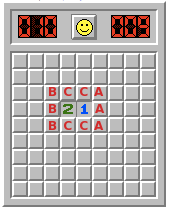Many moons ago, I looked into the game theory of Minesweeper, and - in particular, what you should do in this situation:I think I got my probabilities wrong there, and want to put that right.

If you want to work out the correct move (and associated probabilities), you should read no further than the line.

## Two classes of possibility

There are two broad classes of possibility for what might be behind the squares here: either there are two mines (one in the three left-most squares and two in the four central squares) or there are three (two on the left and one in the three rightmost squares). The first question is, which is more likely?

## Two mines?

To work out the likelihood of the two-mine situation, I’m going to work out a series of probabilities - or rather, count the possibilities:

• there are exactly eight mines in the 69 squares we have no information about
• there is exactly one mine among the three left-most squares
• there is exactly one mine among the three right-most squares

This works out to $\nCr{69}{8} \times \nCr{3}{1} \times \nCr{3}{1}$.

I’m not going to work that out: I’m only after the relative probabilities, so I’ll hopefully be able to work out a ratio and cancel it down.

## Three mines

Here, I need the possibilities:

• there are seven mines in the 69 outer squares
• there are two mines in the three rightmost squares
• there is one mine among the four central squares.

This is $\nCr{69}{7} \times \nCr{3}{2} \times \nCr{4}{1}$.

## The ratio

Writing this out in factorial form, we get the probability ratio (two mines: three mines) to be:

$\frac{69!}{(8!)(61!)} \times 3 \times 3 : \frac{69!}{(7!)(62!)} \times 3 \times 4$.

That simplifies down substantially even at first glance:

$(7!)(62!)(3) : (8!)(61!)(4)$

And yet further if we simplify the factorials:

$62 \times 3 : 8 \times 4$, which is $93:16$.

So, for every 109 times you see this pattern, it’ll be two mines 93 times and three mines 16 times (about 6:1).

## What should you do?

Each of the left-hand mines has a one-in-three chance of being a mine 93 times out of 109, and a two-in-three chance the remaining 16 times. That adds up to $\frac{93}{327}+ \frac{32}{327} = \frac{125}{327}$ - a little under 40%.

The four central squares are clear 16 times in 109, but have a one-in-four chance the remaining 93 times, making a probability of $\frac{93}{436}$, about 21%.

The three right-hand squares are clear 93 times in 109, and have a one-in-three chance the rest of the time, being $\frac{16}{327}$, a shade under 5%.

And we shouldn’t forget the rest of the board: that’s $\frac{93}{109}\times\frac{8}{69} + \frac{16}{109}\times \frac{7}{69}$, or $\frac{744 + 112}{7521}$, which is about $11.4$%.

Your best shot at survival is to click one of the three squares on the right. I hope that opens up a few blanks for you!

* Edited 2019-09-30 to fix a typo (lifelihood).